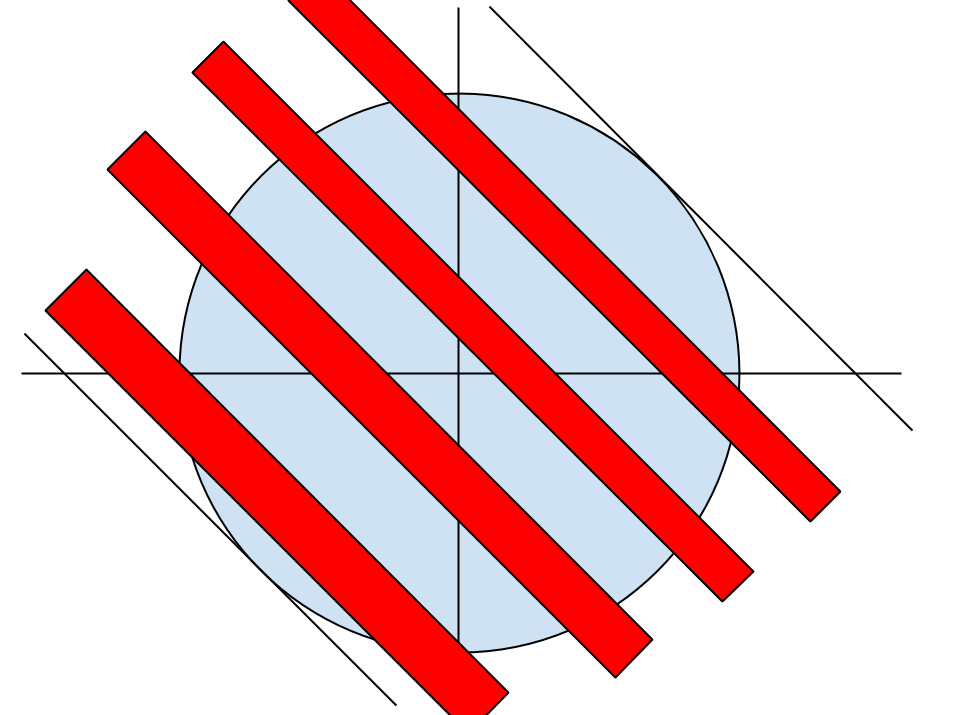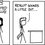# A complicated area problem: between a circle and $\sin(2x + 3y) \le 0$Hi people! I came across this problem while writing an entrance test to CMI (Chennai Mathematical Institute) today. The question is to find the area between the two curves

$\displaystyle x^2 + y^2 = 144$ and $\displaystyle \sin(2x + 3y) \le 0$.

Well, it can be done, definitely, by "brute force" method - what we usually do to find area between two curves. But that would be insanely complicated! So is there a "nice" ("nice" is subjective - I know) way to solve this problem?

Just to share what I started off with:

$\displaystyle \sin(2x + 3y) \le 0$ $\displaystyle \implies (2n+1) \pi \le 2x + 3y \le (2n+2) \pi$ where $n$ is obviously an integer. Also, the tangents to the circle with the slope $-\dfrac{2}{3}$ are $2x + 3y = \pm 12\sqrt{13}$. We are interested in the region between these two tangents. So, to obtain the lower value of $n$, we have:

$\displaystyle (2n_{min} + 1) \pi) \ge -12\sqrt{13} \implies n = -7$

To obtain the upper value of $n$, we have: $\displaystyle (2n_{max} + 2) \pi) \le 12\sqrt{13} \implies n = +5$

So, the area we have to find is that of the intersection of the circle and the "strips" given by the region $\displaystyle \implies (2n+1) \pi \le 2x + 3y \le (2n+2) \pi$, where $n = -7, -6, \ldots, 5$. Let's just expand this to get a better idea:

The "strips" are:

1. $\displaystyle \implies -13 \pi \le 2x + 3y \le -12 \pi$
2. $\displaystyle \implies -11 \pi \le 2x + 3y \le -10 \pi$
3. $\displaystyle \implies -9 \pi \le 2x + 3y \le -8 \pi$
4. $\displaystyle \implies -7 \pi \le 2x + 3y \le -6 \pi$
5. $\displaystyle \implies -5 \pi \le 2x + 3y \le -4 \pi$
6. $\displaystyle \implies -3 \pi \le 2x + 3y \le -2 \pi$
7. $\displaystyle \implies -1 \pi \le 2x + 3y \le 0 \pi$
8. $\displaystyle \implies 1 \pi \le 2x + 3y \le 2 \pi$
9. $\displaystyle \implies 3 \pi \le 2x + 3y \le 4 \pi$
10. $\displaystyle \implies 5 \pi \le 2x + 3y \le 6 \pi$
11. $\displaystyle \implies 7 \pi \le 2x + 3y \le 8 \pi$
12. $\displaystyle \implies 9 \pi \le 2x + 3y \le 10 \pi$
13. $\displaystyle \implies 11 \pi \le 2x + 3y \le 12 \pi$

I think (note: here I don't have any solid justification), that, the area between the circle and strip number #13 (that is, $\displaystyle \implies 11 \pi \le 2x + 3y \le 12 \pi$ ) is the same as the area between the circle and $\displaystyle \implies -12 \pi \le 2x + 3y \le -11 \pi$. My reason to think so: The strips are kind of "symmetrical" about the $6.5^{\text{th}}$ strip. I mean, the half of the $6^{\text{th}}$ strip. So, we can move the strips "above" it, and "place" them in the "gaps" below the strip of symmetry. So by "moving" all the strips above the strip of symmetry, we get a continuous, "thicker" strip, which is easier (relatively) to integrate.

In the end ( if you have read till here :P ), I have to say, I couldn't actually find the area. That's why the question is here!Note by Parth Thakkar
7 years, 2 months ago

This discussion board is a place to discuss our Daily Challenges and the math and science related to those challenges. Explanations are more than just a solution — they should explain the steps and thinking strategies that you used to obtain the solution. Comments should further the discussion of math and science.

When posting on Brilliant:

• Use the emojis to react to an explanation, whether you're congratulating a job well done , or just really confused .
• Ask specific questions about the challenge or the steps in somebody's explanation. Well-posed questions can add a lot to the discussion, but posting "I don't understand!" doesn't help anyone.
• Try to contribute something new to the discussion, whether it is an extension, generalization or other idea related to the challenge.

MarkdownAppears as
*italics* or _italics_ italics
**bold** or __bold__ bold
- bulleted- list
• bulleted
• list
1. numbered2. list
1. numbered
2. list
Note: you must add a full line of space before and after lists for them to show up correctly
paragraph 1paragraph 2

paragraph 1

paragraph 2

[example link](https://brilliant.org)example link
> This is a quote
This is a quote
    # I indented these lines
# 4 spaces, and now they show
# up as a code block.

print "hello world"
# I indented these lines
# 4 spaces, and now they show
# up as a code block.

print "hello world"
MathAppears as
Remember to wrap math in $$ ... $$ or $ ... $ to ensure proper formatting.
2 \times 3 $2 \times 3$
2^{34} $2^{34}$
a_{i-1} $a_{i-1}$
\frac{2}{3} $\frac{2}{3}$
\sqrt{2} $\sqrt{2}$
\sum_{i=1}^3 $\sum_{i=1}^3$
\sin \theta $\sin \theta$
\boxed{123} $\boxed{123}$

Sort by:

Well look at this argument ( I am not writing the complete solution) :

For every point in the circle $(x, y)$ satisfying the given equations, there exists a point $(-x,-y)$ that lies in the circle and doesn't satisfy the equations. And the vice-versa is also true.

So the answer is simply half the circle's area, I.e., $72 \pi$

- 7 years, 2 months ago

This seems too simple (not saying incorrect) to believe! Seriously! I spent so much time over it, typing it here, and writing it in the paper, and here you're dusting it off in one line. Man this is awesome!

- 7 years, 2 months ago

So how did the entrance test go, Parth?

- 7 years, 2 months ago

Not very great. The first part (here I assume you too have written the test, and your age isn't really 19) was very easy. Everything, except a mistake in one problem, seems correct. However, in part B, I could do two questions fully, while I could attempt two others partwise. There's no chance of me getting into it. Surely there are at least 40 people (in about 2000 odd people who took the exam) who could do at least five if not all six!

- 7 years, 2 months ago

Yeah buddy, you got that right, I gave the test too. I couldn't do all full, but did part of the last 5 problems. The mcqs were okay......really 2000 gave the test and atleast 40 people did 5 what about ISI entrance, how did it go??

- 7 years, 2 months ago

2000 is a number I got from quora (some guy had made a rough analysis). Rough figure. And 40 is the roundabout number who'll be getting a seat there. Surely there will be these many people. Obviously! And not to mention there will be a few people who'll be getting direct admission - INMO, RMO guys.

- 7 years, 2 months ago

Yeah you're right, what about ISI entrance? You gave it right?

- 7 years, 2 months ago

No. Wasn't careful at that time.

- 7 years, 2 months ago

And you might not worry about the case when $sin (2x+3y) =0$, because it resembles sets of lines, after all, and wouldn't affect the area. :D

- 7 years, 2 months ago

What were the other questions in that you found interesting?

Staff - 7 years, 2 months ago

Will surely be adding them soon!

- 7 years, 2 months ago

- 7 years, 2 months ago

Here's another: And you thought limits were always easy

I'll post others sometime later. Thanks for showing interest :D

- 7 years, 2 months ago

I couldn't stop myself from posting this one: Polynomials? That sounds familiar

- 7 years, 2 months ago

hey buddy i also wrote the same examination. in the question sin(2x+3y)<=0 you can write it as 2x+3y<=0.since if you take sin inverse on both sides you get the above equation and hence can find the area easily

- 7 years, 2 months ago

Consider the point $(\pi/2, 0)$. Does this satisfy $\sin(2x+3y) \le 0$? Yes. Does it satisfy $2x + 3y \le 0$? No. You can't write that way.

- 7 years, 2 months ago

you can write it that way.if you are that unsure you can check it out in that new pattern iit by arihant. it has the same question but with different data.

- 7 years, 2 months ago

It is wrong my friend! If the book has written that way, it is a grave mistake. It might be so that the answer turns out to be the same - but that is only a huge coincidence. Move the circle to some point other than origin, let's say $50, 50$. Clearly the circle doesn't even touch the line $2x+3y = 0$. So according to you the answer would be $0$. Clearly wrong - since the curve $\sin(2x+3y) \le 0$ is spread all over the $x-y$ plane. I don't know of any software that would plot and show you, (wolframalpha fails too). Think over for a while.

- 7 years, 2 months ago

yup buddy the ans is 72pie, yhe same question with a diff version came in ISI , its just a straight line passing thru (0,0) also the centre.

- 7 years, 1 month ago

I Cleared bothe Cmi & ISI

- 7 years ago